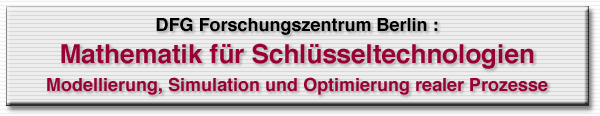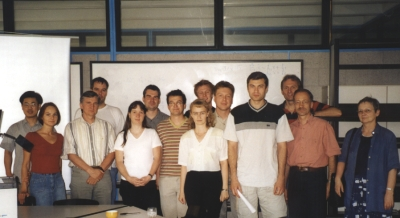## Structure analysis for simulation and control problems of differential algebraic equations

 Duration: August 2002 - May 2006 Project directors: Prof. Dr. R. März, Prof. Dr. V. Mehrmann Department of Mathematics, Humboldt-Universität zu Berlin, Unter den Linden 6, 10099 Berlin, Germany Tel: +49 (0)30 - 2093 2861 (office) / - 2093 2353 (secretary) email: maerz@mathematik.hu-berlin.de Technische Universität Berlin, Institut für Mathematik, Straße des 17. Juni 136, 10623 Berlin, Germany Tel: +49 (0)30 - 314 25736 (office) / - 314 21264 (secretary) email: mehrmann@math.tu-berlin.de Project collaborator: R. Lamour Department of Mathematics, Humboldt-Universität zu Berlin, Unter den Linden 6, 10099 Berlin, Germany Tel: +49 (0)30 - 2093 2630 email: lamour@mathematik.hu-berlin.de Further collaborators: A. Backes Department of Mathematics, Humboldt-Universität zu Berlin, Unter den Linden 6, 10099 Berlin, Germany Tel: +49 (0)30 - 2093 5436 email: a.backes@de.tecosim.com

### Background

The development of efficient and reliable numerical methods for the simulation of large scale differential-algebraic systems (DAEs) that arise in circuit simulation (but also in other applications) is essential for the progress in technology.

In order to cope with further miniaturization one has to deal with parasitic effects like coupling capacities or influences of substratum. This leads to very stiff systems on the order of 1000000 equations, whose differential-index (if defined) may be larger than 2 and which have only moderately smooth coefficients. For such problems it is necessary to develop efficient and reliable index-monitors and diagnostic tools to ensure the stability of numerical integrators and to diagnose bad cases.

In recent years much effort has been spent from different groups to develop a systematic theory as well as appropriate numerical algorithms for DAEs. This theory and the resulting numerical methods such as derivative arrays or geometrical methods are, however, not fully adequate for the current requirements of minimal smoothness of the data and the size and structure of the problems in circuit simulation. Moreover, it has recently been observed that the so-called structural index resulting from the (combinatorial) Pantelides-algorithm is not the right concept for the analysis of DAEs.

### Research program

One of the aims of this project is to bring together two important approaches for the theory of DAEs.

The theory of the tractability-index for DAEs with properly stated leading term was motivated by problems arising in circuit simulation where these structures arise naturally. It uses recursively defined matrix sequences that are based upon appropriate projectors. The tractability index is defined without the use of derivative arrays (e.g. , ,,,) what meets the situation in many applications. The index monitor developed on this basis for the simulator package TITAN (see ) applies to quite large systems. Local index checks can be realized by linear algebra tools (see ). By means of appropriate scalings and projectors, linear DAEs can be decoupled into their characteristic parts (inherent regular ODE, obvious and hidden constraints). This allows for strong solvability statements and new results concerning optimal control problems (, ,,,).

The concept of the strangeness-index uses derivative arrays that contain all the necessary information about the DAE to extract a new system that contains all algebraic constraints plus some differential conditions. This system has the same solution set as the original DAE (see ). Software has been developed to solve linear and nonlinear DAEs of arbitrary index (see , ), and also over- and underdetermined systems can be solved using this theory. This concept also allows the application to control problems with a behaviour approach (e.g. ). Additional structure of certain problems can be used to improve the efficiency of the numerical methods (see ). The theory and the methods have been extended to BVPs (e.g. ).

At this stage it is unclear how the theories of the tractability- and the strangeness-index are related, in particular for problems with high index. For example it is not known if the regularity conditions of the data are equivalent. We realized that any linear DAE having a well-defined regular strangeness index k <= 4 has tractability index k+1. Also, the behaviour of solutions at critical points, such as singularities or those points where characteristical quantities of the DAE change, is under investigation. Particular results concerning the characterization of critical points are obtained in ,and .Regular workshops  are organized to discuss these research topics and recent developments in theory and numerical solution.Participants of the first workshop on differential-algebraic equations

### Cooperation.

We expect the project to benefit from a close cooperation with the projects "Numerical simulation of integrated circuits" (R. März, C. Tischendorf), "Model reduction for large-scale systems in control and circuit simulation" (P. Benner, V. Mehrmann, F. Tröltzsch) and "Solution of large unstructured linear systems in circuit simulation" (V. Mehrmann, M. Bollhöfer).

### References

  R. März, D. Estevez Schwarz, U. Feldmann, S. Sturzel, C. Tischendorf: Finding beneficial DAE structures in circuit simulation. In; W.Jaeger, H.-J. Krebs(Eds): Mathematics - Key Technology for the Future. Springer 2002,412-428  R. März: Differential algebraic systems with properly stated leading term and MNA equations.  In K. Anstreich, R. Bulirsch, A. Gilg, and P. Rentrop, editors, Modelling, Simulation and Optimization of Integrated Circuits, pages 135-151. Birkhäuser, 2003.  R. März: The index of linear differential algebraic equations with properly stated terms. Results Math. 42, 2002,308-338  R. März: Solvability of linear differential algebraic equations with property stated leading terms.  Results Math., 45(1-2):88-105, 2004.  R. März: Fine decouplings of regular differential-algebraic equations. Results Math., 46:57-72, 2004.  S.L. Campbell, R. März: Characterization and computation of a ''good control''. Preprint 04-9, Institut für Mathematik, Humboldt-Universität zu Berlin, 2004.  K. Balla, G. Kurina, R. März: Index criteria for differential algebraic equations arising from linear-quadratic optimal control problems. Technical Report 86, MATHEON, DFG Research Center ``Mathematics for Key Technologies'', 2004.  G.A. Kurina, R. März: On linear-quadratic optimal control problems for time-varying descriptor systems. SIAM J. Control Optim., 42(6):2062-2077, 2004.  G.A. Kurina, R. März: Feedback solutions of optimal control problems with DAE constraints. Preprint 2005-9  R. März: Characterizing differential algebraic equations without the use of derivative arrays. Comput. Math. Appl., 50(7):1141-1156, 2005.  R. März, R. Riaza: Linear index-1 DAEs: regular and singular problems. Acta Appl. Math., 84:29-53, 2004.  R. März, R. Riaza: On linear differential-algebraic equations with properly stated leading term. I. regular points. Preprint 04-22, Institut für Mathematik, Humboldt-Universität zu Berlin  R. März, R. Riaza: On linear differential-algebraic equations with properly stated leading term. II. critical points. Preprint 04-23, Institut für Mathematik, Humboldt-Universität zu Berlin, 2004.  R. Lamour: Index determination and Calculation of Consistent Initial Values for DAEs. Comput. Math. Appl., 50(7):1125-1140, 2005.  P. Kunkel, V. Mehrmann: Analysis und Numerik linearer differentiell-algebraischer Gleichungen, in Wissenschaftliches Rechnen. Eine Einführung in das Scientific Computing, J. Herzberger Herausg., Akademie Verlag Berlin 1995, 233-278.  P. Kunkel, V. Mehrmann: Analysis of over- and underdetermined nonlinear differential-algebraic systems with application to nonlinear control problems. Math. Control Signals Systems 14, 233-256 (2001)  P. Kunkel, V. Mehrmann, W. Rath, J. Weickert: GELDA -- Ein Softwarepaket zur Lösung linearer differentiell-algebraischer Gleichungen mit beliebigem Index. Software Engineering im Scientific Computing, Mackens, Rump (Hrsg.), Vieweg, Braunschweig (1996)  P. Kunkel, V. Mehrmann: Index reduction for differential-algebraic equations by minimal extension. Preprint 719, Institut für Mathematik, TU Berlin (2001)  P. Kunkel, V. Mehrmann, I. Seufer: GENDA: A software package for the numerical solution of GEneral Nonlinear Differential-Algebraic equations. Preprint 730, Institut für Mathematik, TU Berlin (2002)  P. Kunkel, V. Mehrmann, R. Stöver: Symmetric collocation for unstructured nonlinear differential-algebraic of arbitrary index. Preprint 02-12, Zentrum für Technomathematik, Uni Bremen (2002)  A. Backes: Extremalbedingungen für Optimierungsprobleme mit Algebro-Differentialgleichungen. Dissertation, Eingereicht an Humboldt-Universität zu Berlin, 2005